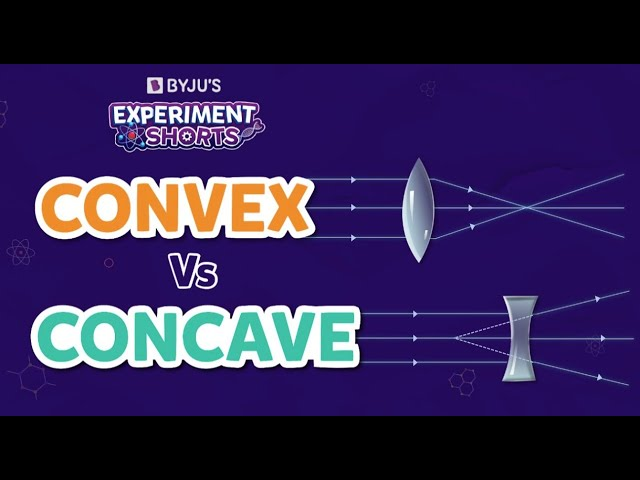# Convex Lens Questions

The lens is an optical medium that converges or diverges light by refraction. A lens can be made using glass or plastic.

Most lenses are spherical, which means their surfaces are parts of the spheres. Each surface can be planar, convex, or concave, as per need.

Convex lenses have thin edges and are thick in the middle. A convex lens is a converging lens since it can bend light rays inwards and converge at the focal length. Convex lenses are mainly used to correct hypermetropia or long-sightedness. They are used in cameras to focus light and produce a clear and crisp image.

Read more: Lenses in optics, Concave and Convex Lens

## Important Convex Lens Questions with Answers

1. Which of the following is a converging lens?

1. Concave lens
2. Convex lens
3. A mirror
4. Planar lens

Explanation: Convex lens is a converging lens. It can converge to a straight beam of light.

2. A biconvex lens features _____.

1. two concave sides
2. two convex sides
3. one concave and one convex side
4. neither concave nor convex side

Explanation: A biconvex lens features two convex sides.

3. When parallel light rays pass through a convex lens, the refracted rays converge at a point known as the _____.

1. focal length
2. focal point
3. focus
4. principal focus

Explanation: When parallel light rays pass through a convex lens, the refracted rays converge at a point known as the principal focus.

4. A prime lens has _____.

1. varying focal lengths
2. fixed focal length
3. both fixed and varying focal lengths
4. neither fixed nor varying focal lengths

Explanation: A prime lens has a fixed focal length, so it does not support zooming in or out.

5. State true or false: Convex lens diverges the beam of light.

1. TRUE
2. FALSE

Explanation: Convex lens does not diverge the light; it converges the light.

6. Which lens is thinner at the centre?

1. Concave lens
2. Convex lens
3. A mirror
4. Planar lens

Explanation: Concave lens is thinner at the centre. It gets thicker as we move towards the edges.

7. The lens in the human eye is _____?

1. Concave lens
2. Convex lens
3. A mirror
4. Planar lens

Explanation: The lens in the human eye is a convex lens.

8. Define plano-convex lens.

A plano-convex lens is an optical lens with a convex and flat surface..

9. Which type of lens is used in a magnifying glass?

1. Concave lens
2. Convex lens
3. A mirror
4. Planar lens

Explanation: Convex lens is used in a magnifying glass.

10. What is the formula to calculate magnification?

Magnification is given by the formula:

Magnification = Image height / Object height

## Practice Questions

1. What is a lens?
2. How are optical lenses classified?
3. What are the differences between concave and convex lenses?
4. List the application of convex lenses.
5. Which lens is used in a telescope?

Wouldn’t it be super cool to just directly visualize ray diagrams? So, how about we make a visual aid to unravel the hidden mystery of light! Watch the video to know more!Stay tuned to BYJU’S and Fall in Love with Learning!